# Class 8 Maths NCERT Solutions for Chapter – 1 Rational Numbers Ex – 1.1

## Rational Numbers

Question 1.
Using appropriate properties find.Solution:Question 2.
Write the additive inverse of each of the following: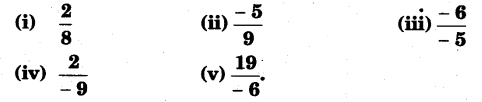Solution: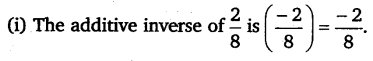Question 3.
Verify that – (-x) = x for:
(i)$x=\frac { 11 }{ 15 }$
(ii)$x=-\frac { 13 }{ 17 }$.

Solution: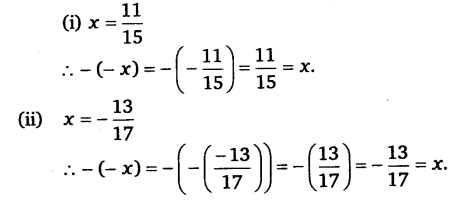Question 4.
Find the multiplicative inverse of the following: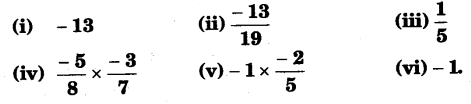Solution: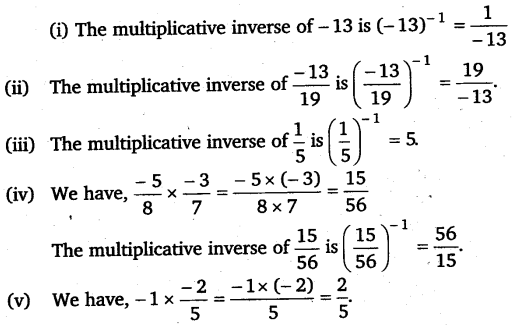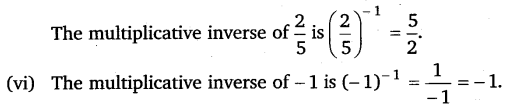Question 5.
Name the property under multiplication used in each of the following: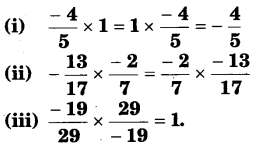Solution:
(i) Existence of multiplicative identity.
(ii) Commutative property of multiplication.
(iii) Existence of multiplicative inverse.

Question 6.
Multiply$\frac { 6 }{ 13 }$ by the reciprocal of$\frac { -7 }{ 16 }$.

Solution: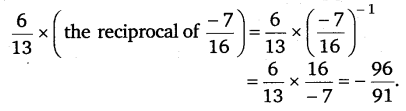Question 7.
Tell what property allows you to compute.$\frac { 1 }{ 3 } \times \left( 6\times \frac { 4 }{ 3 } \right) as\left( \frac { 1 }{ 3 } \times 6 \right) \times \frac { 4 }{ 3 }$
Solution:
Associative property of multiplication over rational numbers allows us to compute :$\frac { 1 }{ 3 } \times \left( 6\times \frac { 4 }{ 3 } \right) as\left( \frac { 1 }{ 3 } \times 6 \right) \times \frac { 4 }{ 3 }$

Question 8.
Is$\frac { 9 }{ 8 }$ the multiplicative inverse of – 1$\frac { 1 }{ 8 }$? Why or why not?

Solution:
No,$\frac { 9 }{ 8 }$ is not the multiplicative inverse of -1$\frac { 1 }{ 8 }$.
Because$\frac { 8 }{ 9 } \times -1\frac { 1 }{ 8 } =\frac { 8 }{ 9 } \times \frac { -9 }{ 8 } =-1\neq 1$.

Question 9.
Is 0.3 the multiplicative inverse of 3$\frac { 1 }{ 3 }$? Why or why not?

Solution:
Yes, 0.3 is multiplicative inverse of 3$\frac { 1 }{ 3 }$.
Because$0.3\times 3\frac { 1 }{ 3 } =\frac { 3 }{ 10 } \times \frac { 10 }{ 3 } =1$.

Question 10.
Write:
(i)
The rational number that does not have a reciprocal.
(ii) The rational numbers that are equal to their reciprocals.
(iii) The rational number that is equal to its negative.

Solution:
(i) We know that there is no rational number which when multiplied with 0, gives 1. Therefore, the rational number 0 has no reciprocal.
(ii) We know that the reciprocal of 1 is 1 and the reciprocal of -1 is -1. 1 and -1 are the only rational numbers which are their own reciprocals.
(iii) The rational number 0 is equal to its negative.

Question 11.
Fill in the blanks :
(i)
Zero has ……………. reciprocal.
(ii) The numbers ……………. and ………. are their own reciprocals.
(iii) The reciprocal of – 5 is …………….
(iv) Reciprocal of$\frac { 1 }{ x }$, where ≠0 is ………
(v) The product of two rational numbers is always a ………….
(vi) The reciprocal of a positive rational number is ………..

Solution:
(i) No,
(ii) 1, -1,
(iii)$\frac { -1 }{ 5 }$
(iv) x,
(v) rational number,
(vi) positive.

### Take Your IIT JEE Coaching to Next Level with Examtube

• Mentoring & Teaching by IITians
• Regular Testing & Analysis
• Preparation for Various Engineering Entrance Exams
• Support for School/Board Exams